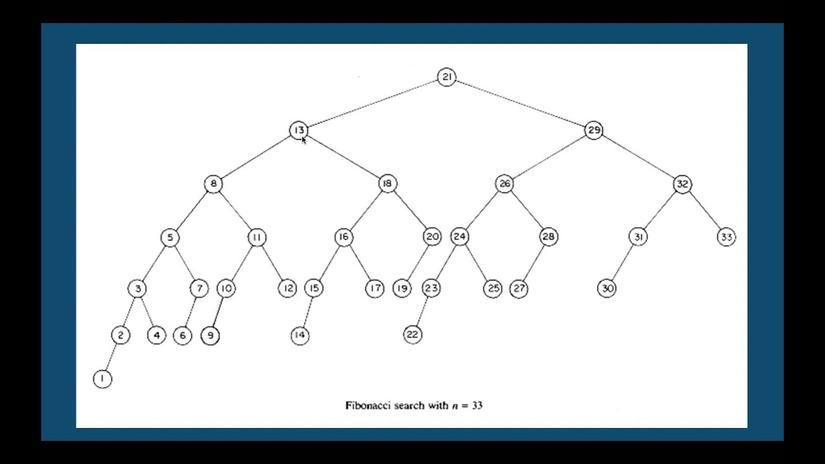# Fibonacci SearchSearching and sorting is always one of the core thing to know and improve about. Like sorting, searching also get special attention from the computer scientists. Today we will learn about a special searching algorithm named fibonacci sort.

### Fibonacci Search

Fibonacci search method is a technique of searching a sorted array using a divide and conquer algorithm that compress the possible places with the help of Fibonacci numbers. In comparison with binary search, in which the sorted array is divided into two equal-sized arrays, one of which is searched further, Fibonacci search divides the array into two parts that have sizes that are consecutive Fibonacci numbers. On an average, this leads to about 4% more comparisons to be executed, although it has the merit that one only needs addition and subtraction to calculate the indices of the accessed array elements. Morever, traditional binary search needs bit-shift, division or multiplication operations that were less common at the time Fibonacci search was first introduced. Fibonacci search has an average- and worst-case complexity of O(log n). To discuss more, The Fibonacci sequence has the property that a number is the sum of its two incestors. Therefore the sequence can be generated by repeated addition. Binary search works by dividing the seek area in equal parts (1:1). Fibonacci search can divide it into parts approaching 1:1.618 while using the simpler operations.

### Algorithm

We assume that the govern sorted array is arr and we want to find element and also we will use the following steps to find the element with minimum steps:

1. At first, search for the smallest Fibonacci number greater than or equal to n. Let this number be fb(M) [m’th Fibonacci number]. Let the two Fibonacci numbers preceding it be fb(M-1) [(m-1)’th Fibonacci number] and fb(M-2) [(m-2)’th Fibonacci number].

2. While the array has elements to be checked:

-> comparison element with the last element of the range covered by fb(M-2)

-> If element equals, return index value

-> Else if element is less than the element, transfer the third Fibonacci variable two Fibonacci down, indicating removal of approximately two-third of the unsearched array.

-> Else element is greater than the element, move the third Fibonacci variable one Fibonacci down. Reset offset to index. Together this results into removal of approximately front one-third of the unsearched array.

3. Since there might be a single element remaining for comparison, check if fbMm1 is '1'. If Yes, compare x with that remaining element. If match, return index value.### Implementation

Implementation in ruby as follows

``````def fibonacci_search int arr, int element
n = n.size
f2 = 0
f1 = 1
f = f2 + f1
offset = -1

while  f < n do
f2 = f1;
f1 = f;
f  = f2 + f1;
end

while f > 1 do
i = [offset+f2, n-1].min

if arr[i] < element
f  = f1
f1 = f2
f2 = f - f1
offset = i
elsif arr[i] > element
f  = f2
f1 = f1 - f2
f2 = f - f1
else
return i
end
end

return offset + 1 if f1 && arr[offset + 1] == element

-1
end
``````

### Complexity

From the above algorithm it is seen that if we have to search the larger section of the array then the runtime will be more and will result into worst case and it's complexity wil be O(log n). If on the very first search, we get our element then it will be considered as the best case and complexcity will be O(1). When we consider the average case then case left and lies between the best and worst i when we have to search the element on the smaller section of the array and hence we get our average case complexity as O(log n). If the element which is being searched have non-uniform access memory storage (i. e., the time needed to access a storage location varies depending on the location accessed), the Fibonacci search may have the advantage over binary search in slightly reducing the average time needed to access a storage location. If the machine executing the search has a direct mapped CPU cache, binary search may lead to more cache misses because the elements that are accessed often tend to gather in only a few cache lines; this is mitigated by splitting the array in parts that do not tend to be powers of two. If the data is stored on a magnetic tape where seek time depends on the current head position, a tradeoff between longer seek time and more comparisons may lead to a search algorithm that is skewed similarly to Fibonacci search.

Hope this helps Thank you Reference: https://iq.opengenus.org/fibonacci-search/